International
Tables for
Crystallography
Volume B
Reciprocal space
Edited by U. Shmueli

International Tables for Crystallography (2006). Vol. B. ch. 2.5, pp. 306-308   | 1 | 2 |

## Section 2.5.4.2. The geometry of ED patterns

B. K. Vainshteinc and B. B. Zvyagind

#### 2.5.4.2. The geometry of ED patterns

| top | pdf |

In HEED, the electron wavelength λ is about 0.05 Å or less. The Ewald sphere with radius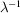has a very small curvature and is approximated by a plane. The ED patterns are, therefore, considered as plane cross sections of the reciprocal lattice (RL) passing normal to the incident beam through the point 000, to scale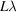(Fig. 2.5.4.1). The basic formula is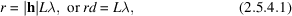where r is the distance from the pattern centre to the reflection, h is the reciprocal-space vector, d is the appropriate interplanar distance and L is the specimen-to-screen distance. The deviation of the Ewald sphere from a plane at distance h from the origin of the coordinates is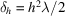. Owing to the small values of λ and to the rapid decrease of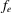depending on, the diffracted beams are concentrated in a small angular interval (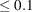rad).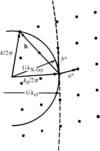Figure 2.5.4.1 | top | pdf |Ewald spheres in reciprocal space. Dotted line: electrons, solid line: X-rays.

Single-crystal ED patterns image one plane of the RL. They can be obtained from thin ideal crystalline plates, mosaic single-crystal films, or, in the RHEED case, from the faces of bulk single crystals. Point ED patterns can be obtained more easily owing to the following factors: the small size of the crystals (increase in the dimension of RL nodes) and mosaicity – the small spread of crystallite orientations in a specimen (tangential tension of the RL nodes). The crystal system, the parameters of the unit cell and the Laue symmetry are determined from point ED patterns; the probable space group is found from extinctions. Point ED patterns may be used for intensity measurements if the kinematic approximation holds true or if the contributions of the dynamic and secondary scattering are not too large.

The indexing of reflections and the unit-cell determination are carried out according to the formulae relating the RL to the DL (direct lattice) (Vainshtein, 1964; Pinsker, 1953; Zvyagin, 1967).

Under electron-diffraction conditions crystals usually show a tendency to lie down on the substrate plane on the most developed face. Let us take this as (001). The vectors a and b are then parallel, while vectoris normal to this plane, and the RL points are considered as being disposed along direct lines parallel to the axiswith constant hk and variable l.

The interpretation of the point patterns as respective RL planes is quite simple in the case of orthogonal lattices. If the lattice is triclinic or monoclinic the pattern of the crystal in the position with the face (001) normal to the incident beam does not have to contain hk0 reflections with non-zero h and k because, in general, the planes ab and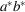do not coincide. However, the intersection traces of direct lines hk with the plane normal to them (plane ab) always form a net with periods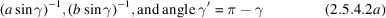(Fig. 2.5.4.2). The points hkl along these directions hk are at distances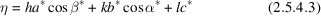from the ab plane.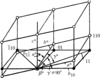Figure 2.5.4.2 | top | pdf |Triclinic reciprocal lattice. Points: open circles, projection net: black circles.

By changing the crystal orientation it is possible to obtain an image of theplane containing hk0 reflections, or of other RL planes, with the exception of planes making a small angle with the axis.

In the general case of an arbitrary crystal orientation, the pattern is considered as a plane section of the system of directions hk which makes an angle ϕ with the plane ab, intersecting it along a direction [uv]. It is described by two periods along directions 0h, 0k;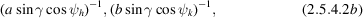with an angle γ″ between them satisfying the relation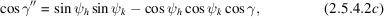and by a system of parallel directions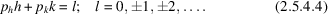The angles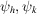are formed by directions 0h, 0k in the plane of the pattern with the plane ab. The coefficients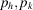depend on the unit-cell parameters, angle ϕ and direction [uv]. These relations are used for the indexing of reflections revealed near the integer positions hkl in the pattern and for unit-cell calculations (Vainshtein, 1964; Zvyagin, 1967; Zvyagin et al., 1979).

In RED patterns obtained with an incident beam nearly parallel to the plane ab one can reveal all the RL planes passing throughwhich become normal to the beam at different azimuthal orientations of the crystal.

With the increase of the thickness of crystals (see below, Chapter 5.1) the scattering becomes dynamical and Kikuchi lines and bands appear. Kikuchi ED patterns are used for the estimation of the degree of perfection of the structure of the surface layers of single crystals for specimen orientation in HREM (IT C, 2004, Section 4.3.8). Patterns obtained with a convergent beam contain Kossel lines and are used for determining the symmetry of objects under investigation (see Section 5.1.2).

Texture ED patterns are a widely used kind of ED pattern (Pinsker, 1953; Vainshtein, 1964; Zvyagin, 1967). Textured specimens are prepared by substance precipitation on the substrate, from solutions and suspensions, or from gas phase in vacuum. The microcrystals are found to be oriented with a common (developed) face parallel to the substrate, but they have random azimuthal orientations. Correspondingly, the RL also takes random azimuthal orientations, havingas the common axis, i.e. it is a rotational body of the point RL of a single crystal. Thus, the ED patterns from textures bear a resemblance, from the viewpoint of their geometry, to X-ray rotation patterns, but they are less complicated, since they represent a plane cross section of reciprocal space.

If the crystallites are oriented by the plane (hkl), then the axis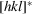is the texture axis. For the sake of simplicity, let us assume that the basic plane is the plane (001) containing the axes a and b, so that the texture axis is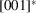, i.e. the axis. The matrices of appropriate transformations will define a transition to the general case (see IT A , 2005). The RL directions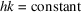, parallel to the texture axis, transform to cylindrical surfaces, the points with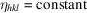are in planes perpendicular to the texture axis, while any `tilted' lines transform to cones or hyperboloids of rotation. Each point hkl transforms to a ring lying on these surfaces. In practice, owing to a certain spread ofaxes of single crystals, the rings are blurred into small band sections of a spherical surface with the centre at the point 000; the oblique cross section of such bands produces reflections in the form of arcs. The main interference curves for texture patterns are ellipses imaging oblique plane cross sections of the cylinders hk (Fig. 2.5.4.3).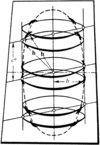Figure 2.5.4.3 | top | pdf |Formation of ellipses on an electron-diffraction pattern from an oblique texture.

At the normal electron-beam incidence (tilting angle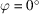) the ED pattern represents a cross section of cylinders perpendicular to the axis, i.e. a system of rings.

On tilting the specimen to an angle ϕ with respect to its normal position (usually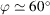) the patterns image an oblique cross section of the cylindrical RL, and are called oblique-texture (OT) ED patterns. The ellipses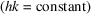and layer lines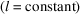for orthogonal lattices are the main characteristic lines of ED patterns along which the reflections are arranged. The shortcoming of oblique-texture ED patterns is the absence of reflections lying inside the cone formed by rotation of the straight line coming from the point 000 at an angle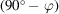around the axisand, in particular, of reflections 00l. However, at ϕ ≲ 60–70° the set of reflections is usually sufficient for structural determination.

For unit-cell determination and reflection indexing the values d (i.e.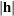) are used, and the reflection positions defined by the ellipses hk to which they belong and the values η are considered. The periods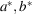are obtained directly from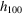and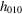values. The period, if it is normal to the plane(being arbitrary), is calculated as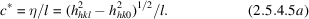For oblique-angled lattices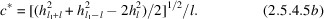In the general case of oblique-angled lattices the coaxial cylinders hk have radii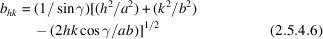and it is always possible to use the measured or calculated values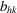in (2.5.4.5a)instead of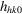, since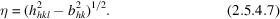In OT patterns theand η values are represented by the lengths of the small axes of the ellipses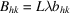and the distances of the reflections hkl from the line of small axes (equatorial line of the pattern)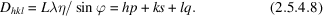Analysis of the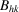values gives a, b, γ, while p, s and q are calculated from the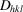values. It is essential that the components of the normal projections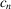of the axis c on the plane ab measured in the units of a and b are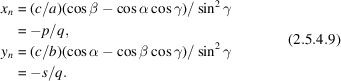Obtaining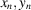one can calculate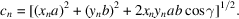Since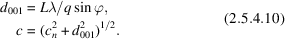The α, β values are then defined by the relations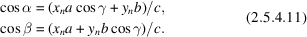Because of the small particle dimensions in textured specimens, the kinematic approximation is more reliable for OT patterns, enabling a more precise calculation of the structure amplitudes from the intensities of reflections.

Polycrystal ED patterns. In this case, the RL is a set of concentric spheres with radii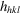. The ED pattern, like an X-ray powder pattern, is a set of rings with radii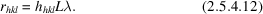### ReferencesInternational Tables for Crystallography (2005). Vol. A. Space-group symmetry, edited by Th. Hahn, 5th ed. Heidelberg: Springer.Google ScholarInternational Tables for Crystallography (2004). Vol. C. Mathematical, physical and chemical tables, edited E. Prince, 3rd ed. Dordrecht: Kluwer Academic Publishers.Google ScholarPinsker, Z. G. (1953). Electron diffraction. London: Butterworth.Google ScholarVainshtein, B. K. (1964). Structure analysis by electron diffraction. Oxford: Pergamon Press.Google ScholarZvyagin, B. B. (1967). Electron-diffraction analysis of clay mineral structures. New York: Plenum.Google ScholarZvyagin, B. B., Vrublevskaya, Z. V., Zhukhlistov, A. P., Sidorenko, S. V., Soboleva, A. F. & Fedotov, A. F. (1979). High-voltage electron diffraction investigations of layered minerals. Moscow: Nauka. (In Russian).Google Scholar